Open in App
Not now

# Esquisse Package in R Programming

• Last Updated : 08 Dec, 2021

Packages in the R programming are a collection of R functions, compiled code, and sample data. They are stored under a directory called “library” in the R environment. By default, R installs a set of packages during installation. One of the most important packages in R is the Esquisse package. Esquisse package helps to explore and visualize your data interactively. It is a Shiny gadget to create ggplot charts interactively with drag-and-drop to map your variables. One can quickly visualize the data accordingly to their type, export to ‘PNG’ or ‘PowerPoint’, and retrieve the code to reproduce the chart.

### Installation

To use a package in R programming one must have to install the package first. This task can be done using the command install.packages(“packagename”).

```source("https://install-github.me/dreamRs/esquisse")
install.packages("esquisse")```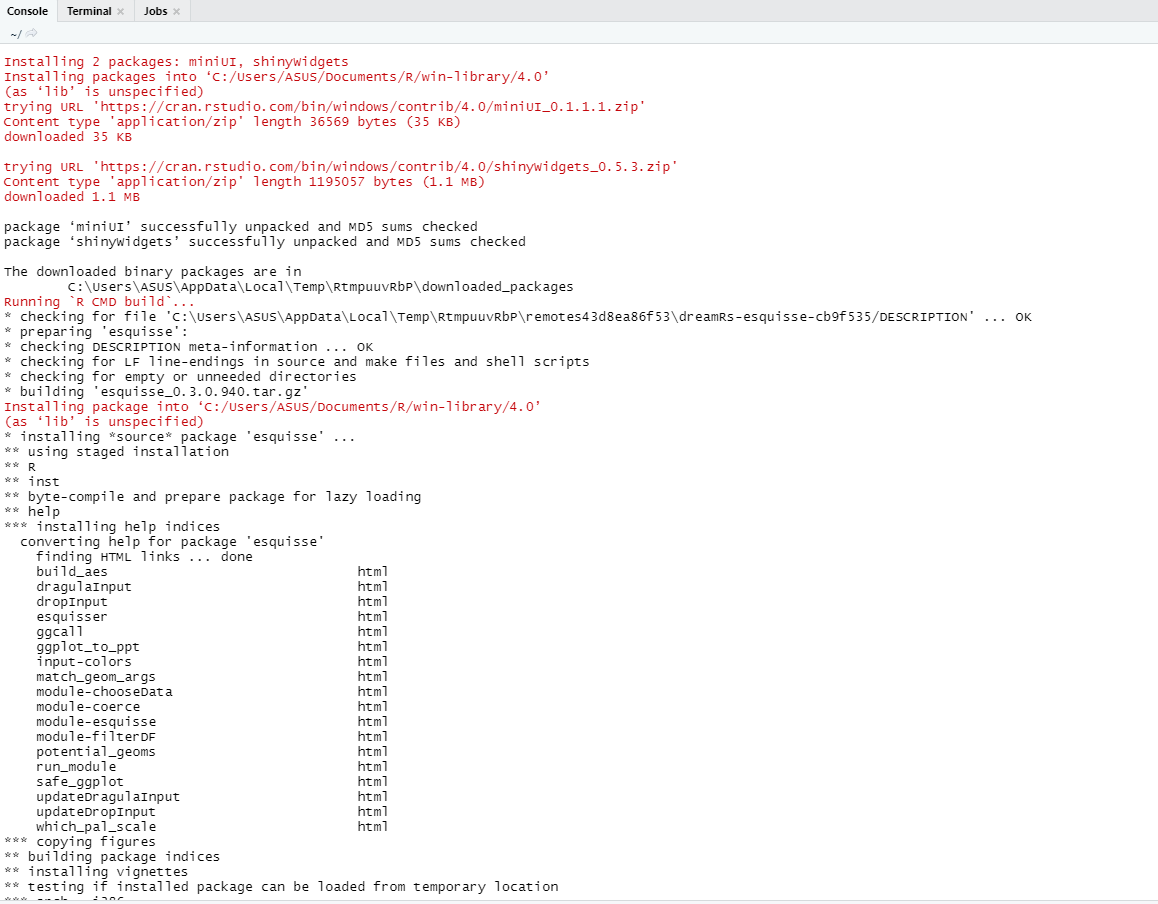To install the development version from GitHub type this:

```# or with devtools:
devtools::install_github("dreamRs/esquisse")```

### Important Verb Functions in Esquisse Package

• chooseData-Module: It is a module for choosing data.frame from the user environment and select variable to use. It gives the user an option to choose from a given list of available datasets to work on within a shiny app.

Syntax:

chooseDataUI(id)

chooseDataServer(input, output, session, data = NULL, name = NULL,

selectVars = TRUE, launchOnStart = TRUE, defaultData = NULL)

Example:

## R

 `# Import shiny and` `# esquisse packages` `library``(shiny)` `library``(esquisse)`   `ui <- ``fluidPage``(` `  ``tags\$``h2``(``"Choose data module"``),` `  ``fluidRow``(` `    ``column``(` `      ``width = 4, tags\$``h4``(``"Default"``),` `      ``# Using chooseDataUI       ` `      ``chooseDataUI``(id = ``"choose1"``),` `      ``verbatimTextOutput``(outputId = ``"res1"``))))`   `server <- ``function``(input, output, session) ` `{` `  ``res_dat1 <- ``callModule``(` `    ``chooseDataServer, id = ``"choose1"``,` `    ``launchOnStart = ``FALSE``)` `  ``output\$res1 <- ``renderPrint``({` `    ``str``(``reactiveValuesToList``(res_dat1))})` `}`   `shinyApp``(ui,server)`

Output: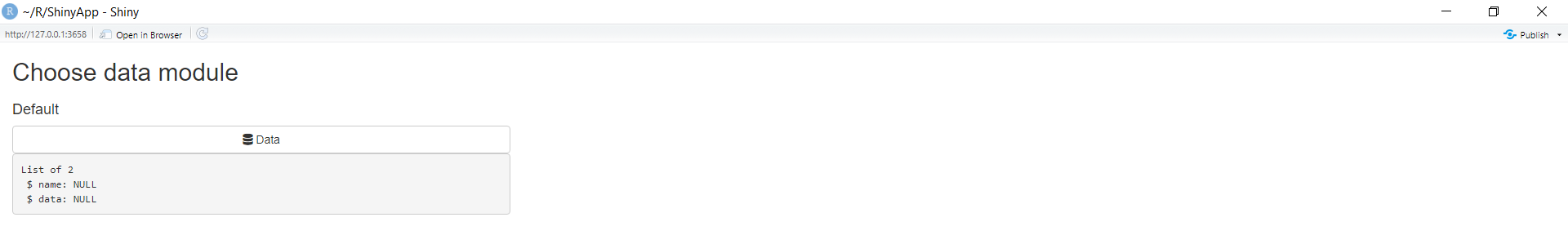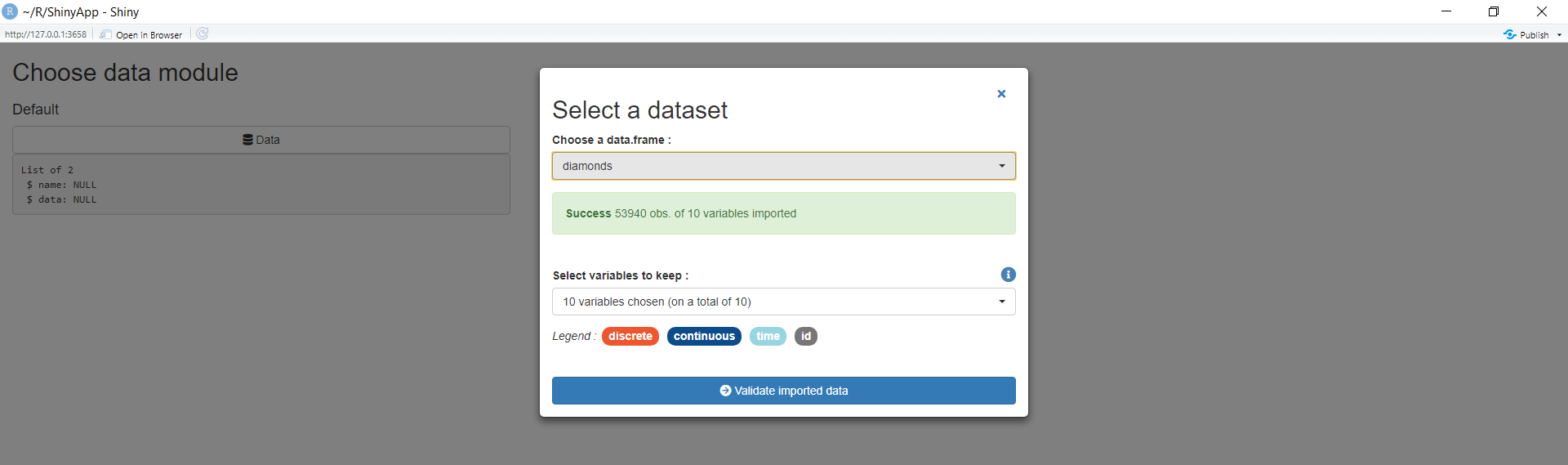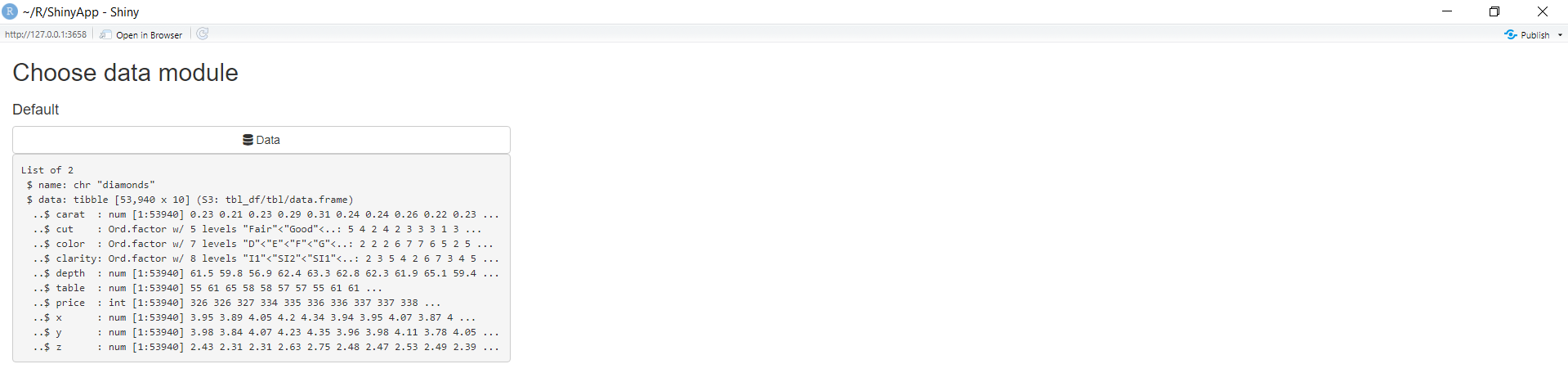• dragulaInput: It creates a Drag And Drop Input Widget. One can select different labels(data) from a variety of labels provided by the developer to the app user to twerk with a simple drag and drop layouts.

Syntax: dragulaInput(inputId, sourceLabel, targetsLabels, targetsIds = NULL,

choices = NULL, choiceNames = NULL, choiceValues = NULL,

status = “primary”, replace = FALSE, badge = TRUE, width = NULL,

height = “200px”)

Example:

## R

 `# Import shiny and` `# esquisse packages` `library``(``"shiny"``)` `library``(``"esquisse"``)`   `ui <- ``fluidPage``(` `  ``tags\$``h2``(``"dragulaInput demo for geeksforgeeks"``),` `  ``tags\$``br``(),` `  ``# using dragulaInput()` `  ``# to create a drag and` `  ``# drop widget` `  ``dragulaInput``(` `    ``inputId = ``"data_di"``, sourceLabel = ``"Source"``,` `    ``targetsLabels = ``c``(``"Drop Here"``, ``"Drop Here 2"``),` `    ``choices = ``names``(rock), width = ``"400px"``),` `    ``verbatimTextOutput``(outputId = ``"result"``))`   `server <- ``function``(input, output, session) ` `{` `  ``output\$result <- ``renderPrint``(``str``(input\$data_di))` `}`   `shinyApp``(ui = ui, server = server)`

Output: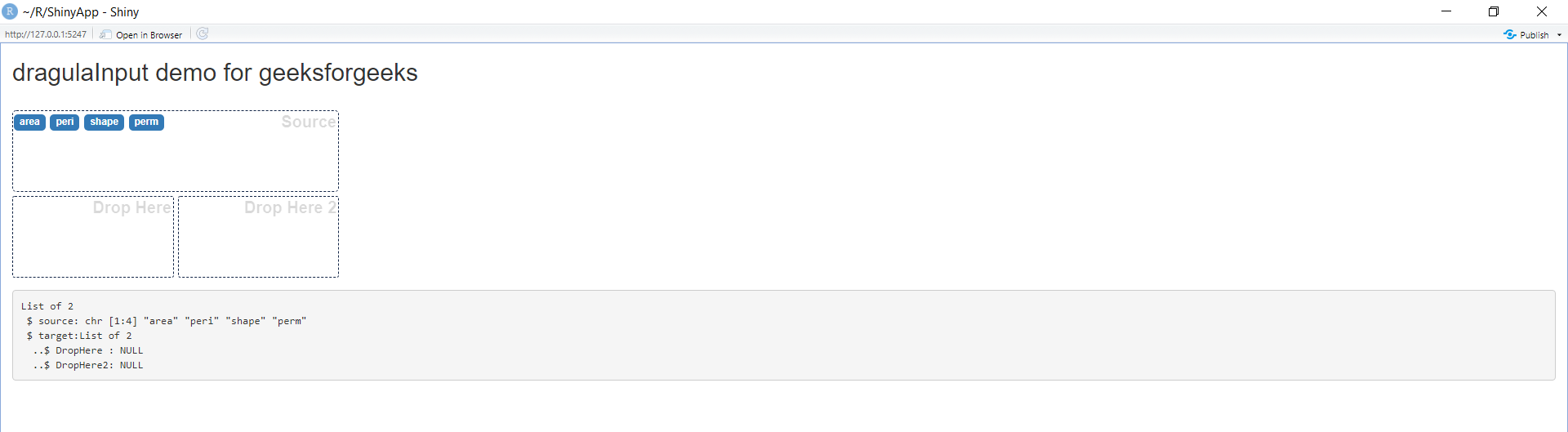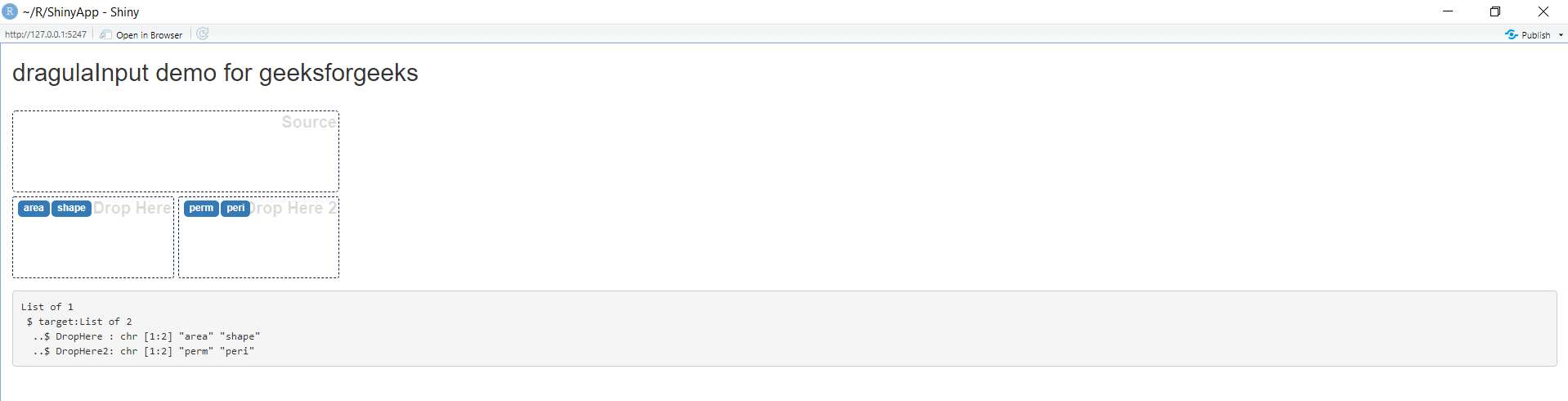• esquisser: It is an add-in to easily create plots with ggplot2. ggplot2 is a system for declaratively creating graphics. Just provide the data, tell ggplot2 how to map variables to aesthetics, what graphical primitives to use, and it takes handles rest all on its own.

Syntax: esquisser(data = NULL)

Example:

## R

 `# Import shiny and` `# esquisse packages` `library``(``"shiny"``)` `library``(``"esquisse"``)` `  `  `esquisser``(rock)`

Output: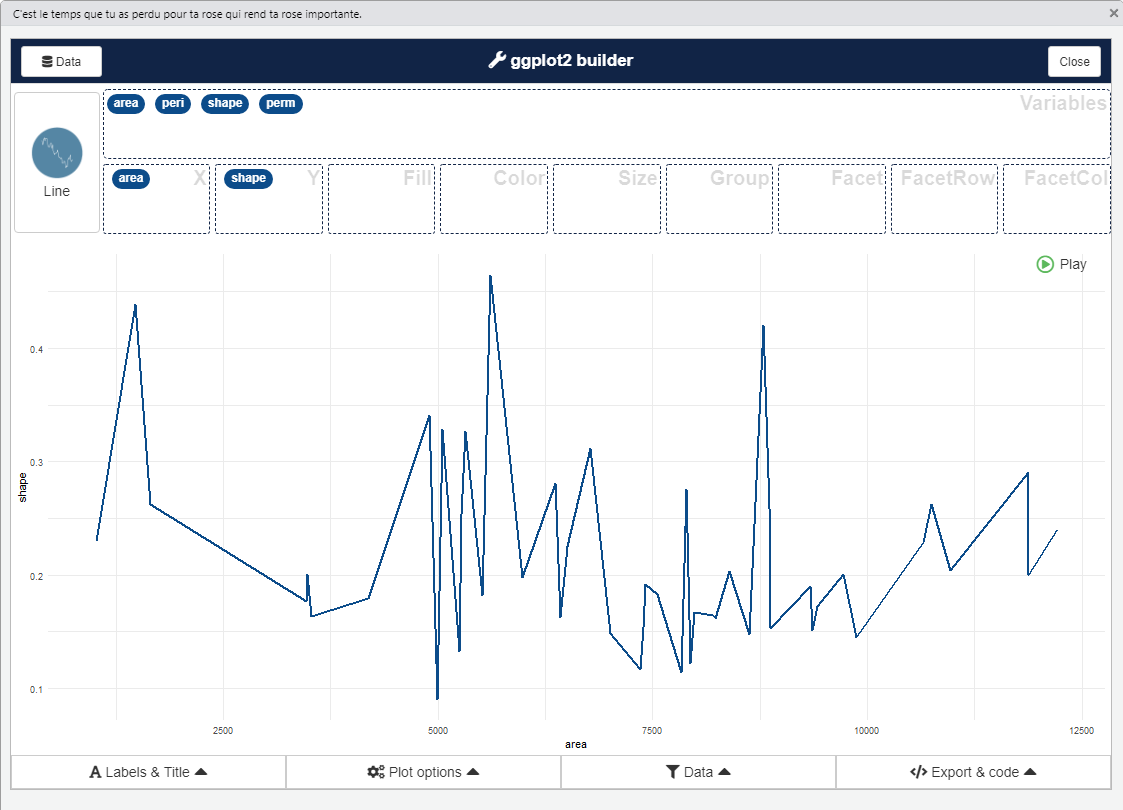• updateDragulaInput: Update Dragula Input. It updates the drag and drop widgets as soon as the call is passed to the function. For instance, if a label is dragged and dropped from the input, the function updates the set of provided input values in the output.

Syntax: updateDragulaInput(session, inputId, choices = NULL, choiceNames = NULL,

choiceValues = NULL, badge = TRUE, status = “primary”)

Example:

## R

 `# Import shiny and` `# esquisse packages` `library``(``"shiny"``)` `library``(``"esquisse"``)`   `ui <- ``fluidPage``(` `  ``tags\$``h2``(``"GfG demo for Update dragulaInput"``),` `  ``radioButtons``(` `    ``inputId = ``"update"``, label = ``"Dataset"``,` `    ``choices = ``c``(``"iris"``, ``"rock"``)),` `  ``tags\$``br``(),` `  ``dragulaInput``(` `    ``inputId = ``"data"``, sourceLabel = ``"Variables"``,` `    ``targetsLabels = ``c``(``"X"``, ``"Y"``, ``"fill"``, ``"color"``, ``"size"``),` `    ``choices = ``names``(iris), replace = ``TRUE``,` `    ``width = ``"400px"``, status = ``"success"``),` `    ``verbatimTextOutput``(outputId = ``"result"``))`   `server <- ``function``(input, output, session) ` `{` `  ``output\$result <- ``renderPrint``(``str``(input\$data))` `  ``observeEvent``(input\$update, {` `    ``if ``(input\$update == ``"iris"``) ` `    ``{` `      ``updateDragulaInput``(` `        ``session = session, inputId = ``"data"``, ` `        ``choices = ``names``(iris), status = ``"success"``)` `    ``} ` `    ``else` `    ``{` `      ``updateDragulaInput``(` `        ``session = session, inputId = ``"data"``, ` `        ``choices = ``names``(rock))` `    ``}` `  ``}, ignoreInit = ``TRUE``)` `  `  `}`   `shinyApp``(ui, server)`

Output: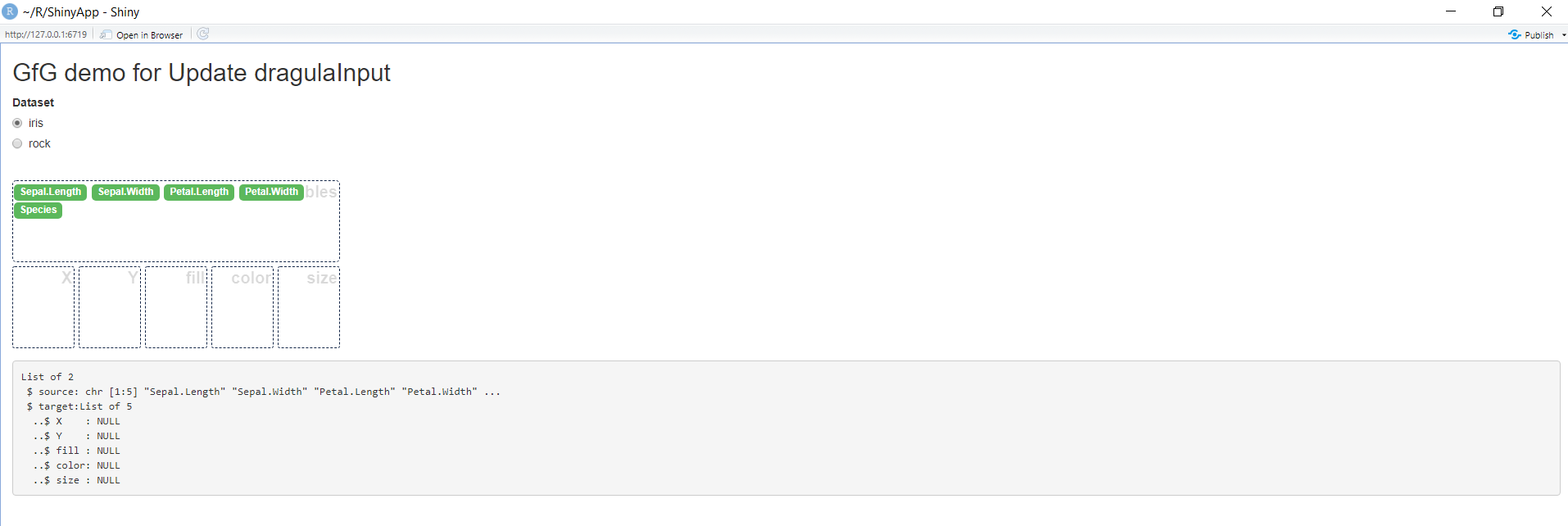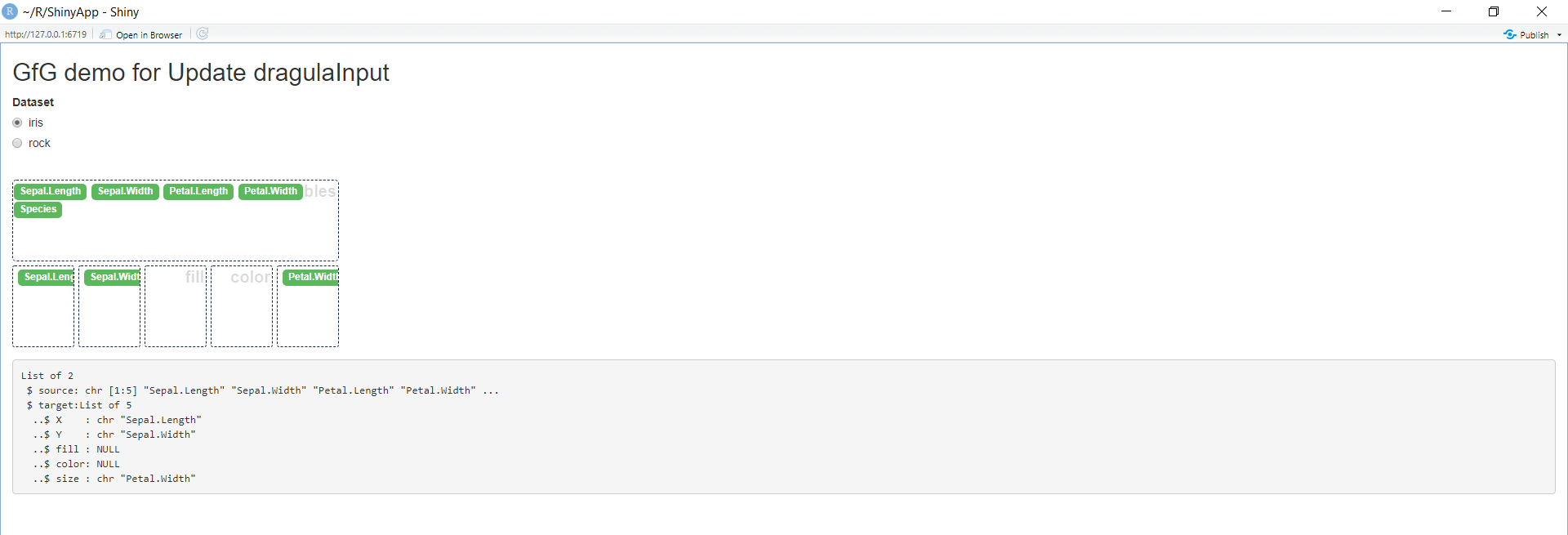• ggplot_to_plot: Utility To Export ggplot Objects To PowerPoint. This utility function provides an easy way to save graphs and models layed using ggplot to a .ppt file or simply a PowerPoint presentation.

Syntax: ggplot_to_ppt(gg = NULL)

Example:

## R

 `# import ggplot2 library` `library``(ggplot2)` `p <- ``ggplot``(iris) + ` `     ``geom_point``(``aes``(Sepal.Length, Sepal.Width))`   `# use ggplot_to_plot` `# to display plot ` `# in a ppt format` `ggplot_to_ppt``(``"p"``)`

Output: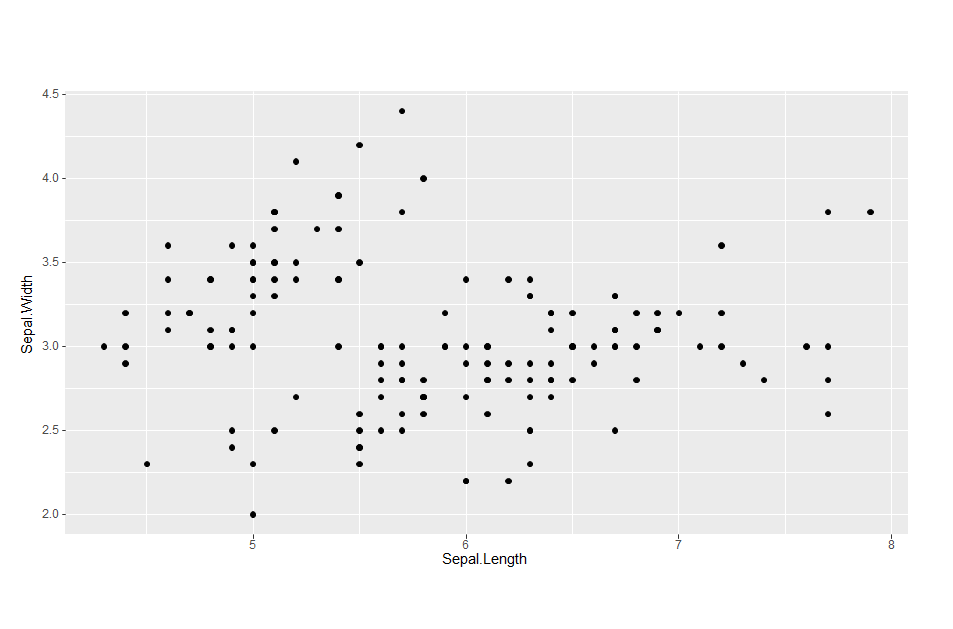The code displays the output in a ppt format. Output to the above piece of code can be seen via this link.

My Personal Notes arrow_drop_up
Related Articles